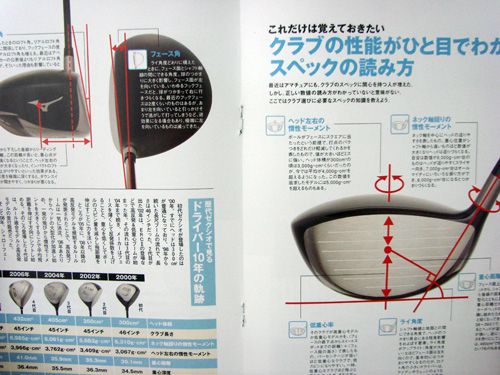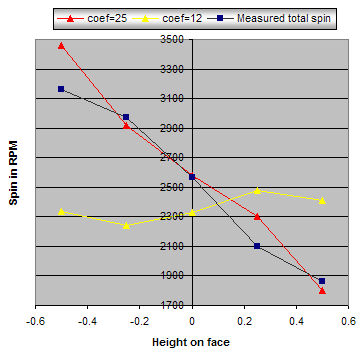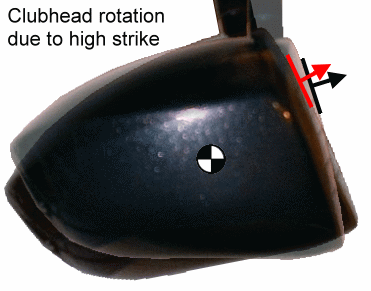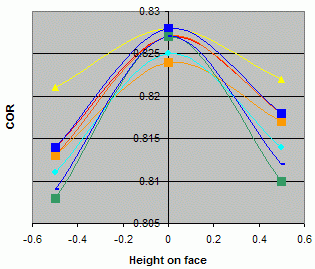# All about Gear Effect - Appendix

Dave Tutelman  --  April 2, 2009

## App 1 - Alba test data from inpakuto.com

This table is a transcription of late-2008 test data from a Japanese web site.

 Club Listed loft Actual loft Horizontal MOI MOI about shaft centerline Depth of CG Shaft centerline to CG Face angle Bridgestone/Tourstage 2009 X-Drive 701 9.5º 10.3º 4192gcm2 7023gcm2 35.3mm 40.8mm Open 0.3º Bridgestone/Tourstage 2009 X-Drive GR 9.5º 10.5º 4351gcm2 7379gcm2 36.3mm 40.0mm Closed 1.0º Srixon ZR-800 9.5º 10.6º 4226gcm2 6794gcm2 34.3mm 40.0mm Square Srixon ZR-30 9.5º 10.5º 3870gcm2 6206gcm2 32.2mm 38.4mm Open 0.5º Mizuno 2009 MP Craft T1 9.5º 9.4º 4241gcm2 6959gcm2 34.1mm 39.4mm Open 0.5º Mizuno 2009 MP Craft R1: 9.5º 10.1º 4527gcm2 7389gcm2 38.2mm 38.9mm Square Titleist 909D2 9.5º 8.7º 4779gcm2 8058gcm2 39.1mm 40.4mm Open 1.0º Titleist 909D3 9.5º 9.8º 4500gcm2 7453gcm2 36.3mm 40.4mm Open 0.5º Taylormade Japan 2009 Burner Plus 10.5º 11.5º 5146gcm2 8479gcm2 42.0mm 40.7mm Closed 1.5º Taylormade Tour Burner 9.5º 10.0º 4821gcm2 8010gcm2 40.0mm 40.3mm Open 1.0º Callaway Legacy 9.5º 9.2º 4204gcm2 7157gcm2 40.5mm 36.8mm Open 1.0º Callaway Hyper X 10.0º 11.3º 4519gcm2 6751gcm2 38.9mm 35.5mm Square Yamaha Inpres X 4.6D 10.0º 12.0º 4339gcm2 6472gcm2 34.6mm 34.9mm Closed 1.0º Nike Sasquatch Sumo2 5900 10.5º 11.7º 5867gcm2 9799gcm2 47.0mm 42.2mm Closed 1.2º Ping Rapture V2 10.5º 12.5º 4478gcm2 7921gcm2 38.0mm 44.1mm Closed 1.0º Cobra L5V 10.5º 14.0º 4875gcm2 7857gcm2 38.4mm 43.2mm Closed 2.0º Cleveland Launcher 10.5º 12.3º 4467gcm2 7968gcm2 37.9mm 43.0mm Closed 1.5ºThis page from Alba magazine shows the key measurements taken for the tests.

## App 2 - Calculations for horizontal gear effect

 Miss (how far towards toe) Horizontal "loft" due to bulge Horizontal "launch angle" Slice spin due to bulge Hook spin due to gear effect Hook spin (net) Total side error Hook without bulge ¼ 1.2° 1.1° 338 553 215 3 yards left 20 yards left ½" 2.4° 2.1° 676 1113 437 6 yards left 38 yards left 1" 4.8° 4.2° 1354 2192 838 10 yards left 61 yards left 1½" 7.1° 6.2° 2005 3294 1289 11 yards left 72 yards left

How the calculations were done, column by column:
 Miss Given Horizontal "loft" Trig: arctan (miss/radius) Horizontal "launch angle" TrajectoWare Drive (TWD): Use horizontal loft as face angle, and read launch angle Slice spin (bulge) TWD: As above, read sidespin Hook spin (gear effect) Compute y from miss and horizontal launch angle: y=miss-Csin(HorLaunchAngle) (See section on vertical gear effect for rationale) Then equation 2a to get the spin. Hook spin (net) The difference of the previous two columns Total side error TWD:Using zero-miss launch conditions, crank in horizontal launch angle and net spin Hook without bulge Use equation 2a for the spin, with y=miss Then TWD to find the size of the hook.

## App 3 -Bend of a shaft loaded with both force and moment

This is a sidebar to the article on Gear Effect. We need to know the details of shaft bend (deflection curve and tip angle) for a cantilever shaft that is loaded with both:
• The force from impact with a golf ball.
• A moment because the impact is y inches above or below the club's center of gravity.
Structures textbooks and web sites have well-known formulas, where L is the beam length and EI is the shaft stiffness:

Deflection D as a function of postion (x) on shaft Angle θ (actually slope) at tip
Applied force
P
 Px2 6EI (3L - x)
 PL2 2EI
Applied moment
M
 Mx2 2EI
 ML EI

If we apply a force that wants to bend the tip backwards (negative) at the same time as a moment that wants to bend the tip forward (positive), then superposition tells us that D and θ are the difference of the Ds and θs for the moment minus the force.
D =
 Mx2 2EI - Px2 6EI (3L - x)
=
 x2 2EI [ M - P(L - x/3)]
θ =
 ML EI - PL2 2EI
=
 L EI ( M - PL/2 )
So far, so good. But we can easily compute what M and P are. They are produced by the ball hitting the clubhead. We encountered them as we derived Equation 1 in the article on gear effect.

Let's do the force first. We know from the impulse-momentum conservation at impact:
mVb  =  F(t)dt
If we take P to be the average force over a half-millisecond impact (.0005 sec), this is just:
mVb  =  .0005 P
Solving for P, we get:
P  =  2000 mVb
Since all golf balls are almost exactly 46 grams (.046Kg):
P  =  92 Vb
The moment is the force times the lever arm y. Looking at the average moment:
M  =  92 Vb y
If we plug these back into the equations for deflection and angle, we get:
 Deflection at x = 46Vb EI x2( y + x/3 - L )
 Tip Angle  = 92Vb EI L( y - L/2 )
These are all metric (MKS) units. We would like to have L, x, y, and Deflection in inches, and Vb in miles per hour. Moreover, the tip angle is in radians, and we want it in degrees. Applying the proper conversion factors:
Deflection at x =
 46 Vb * .447 EI x2( y + x/3 - L ) * .02543 / .0254
=
 .0133 Vb EI x2( y + x/3 - L )
Tip Angle =
 92 Vb * .447 EI L( y - L/2 ) * .02542 * 180 / π

=
 1.52 Vb EI L( y - L/2 )
These are the equations we use in the article.

## App 4 - Modify the model to accommodate GRT

Here are the details of the factor-by-factor re-evaluation of the model, in an attempt to make GRT look like a good design choice.

### Change the parameters of the model

The basis of the model is equation 3a:
spin  =  25 y Vb
That, and the value of C (which we set at 1.3, but could realistically vary between 1.1 and 1.5), are the major contributors to the way the model fits the published data. (C figures in because it is instrumental in determining y in equation 3a.) The simplest, and undoubtedly the most effective, way to vary the model is to play with the value 25 -- the coefficient in equation 3a, so we'll refer to it as coef -- and with the value of C.

I played with C and especially coef until the model gave an optimum roll that was close to GRT.

 Original Model coef=25 C=1.2 Modified Model coef=12 C=1.1 Actual GRTFigure A4-1 Optimum high-face roll 8" 16.4" 15" Optimum low-face roll 10" 19.1" 20"Figure A4-2
This is a good match to the actual face roll of a GRT clubhead. The next question becomes: How does this modified model fare against the test data for vertical gear effect. Let's compare it with the Hotstix data.

Let's review what we are doing here. We have modified the parameters of the mathematical model so that the optimal face roll is essentially the same as GRT. This gives us a new model -- an as-yet unvalidated model. We have data that we can use to validate any model for vertical gear effect. We just have to plug the launch angle and clubhead speed into the mathematical model, and see what backspin we wind up with. If the backspin matches the measured data, then the model is valid; if not, the model is not a good representation of reality.

So let's try it with the new, modified model (coef=12, to match GRT). Figure A4-2 is the comparison. The modified model (yellow line) does not match Hotstix data at all, while the original model (red line) is a good match. The yellow line does not even show backspin decreasing with the height of impact; it is nearly constant -- and increasing if you look closely. So it cannot possibly be a valid model.

And, if it is not a good model for vertical gear effect, it cannot be used to justify GRT. We will have to look elsewhere.

### Shaft tip flex

Suppose shaft tip flex limited the gear effect. If it limited it enough, then GRT might be a superior face roll design. Let's explore this a little. Points to consider:
• The Hotstix test data agrees with the original model, unfettered by shaft stiffness. So, for this assumption to be tweaked enough to make GRT superior, we would need to assume that Hotstix used a flexible-tip shaft on the driver, but GRT users have to use a much stiffer tip.
• Therefore, it would require one of two assumptions:
• That Hotstix took 100mph clubhead-speed data with a shaft ill-fit for most 100mph clubhead-speed golfers. That is not very likely. Hotstix is a competent organization, whose raison d'être is clubfitting.
• That golfers using a GRT clubhead would use a shaft that was much more tip-stiff than normal. I can think of no sound clubfitting reason for this to be the case.
• How much stiffer, and how much good would it do? Even if it were the stiffest you ever tried to use, it would still only cut gear effect by 15%. (We looked at this a few pages ago.) In order to get to the range where GRT works, we need to cut gear effect by 50%. So even a hugely tip-stiff shaft would get us less than a third of what we need.
If we assume that a GRT user will probably want a shaft not wildly stiffer than Hotstix used, even the 15% is way more than we would see in reality. Think about it; do you want to use a shaft that is otherwise too tip-stiff for you, just so that GRT will work for you? If course not! You want a shaft that fits you, not a shaft that allows you to fit a particular face roll profile. So this would work only for a golfer who naturally needs a shaft whose tip is a lot stiffer than normal for his clubhead speed. Bearing that in mind, the limit is probably well under 5%, nowhere near the 15% theoretical maximum.

What would be the influence on roll of a 5% reduction of gear effect due to shaft tip stiffness? (We're being very generous here; there are few golfers that would benefit from a shaft change of this magnitude.)  When we go through re-optimization, we find that:
• At -0.8", the ideal loft goes from 4.7° to 4.9°, a difference of +0.2°.
• At +0.8", the ideal loft goes from 16.0° to 15.6, a difference -0.4°.
• At +0.23", there is no gear effect spin; the ideal loft remains at 11.7°.
Coincidentally, the zero-gear-effect height divides the face quite well into top third and bottom two thirds. So we will be able to see the effect on roll in the zones that GRT divides the face.

### Our formula for backspin due to loft

I am using a formula to go from effective loft to backspin. The formula is not from basic physics by rather a simple equation (second degree polynomial) that gives a good fit to data from several sources. (Cochran & Stobbs' book, the TWGT trajectory profiler, and Max Dupilka's trajectory model program.) It is possible that the sources are incorrect. Alternatively, it is possible that they are correct, but based on measured backspin -- with the gear effect spin already factored in.

The backspin formula is not part of the model of gear effect. But it is (a) part of the calculations by which we validate the model against real-world data, and (b) part of the calculations to optimize face roll. So an error here would cast doubt on both the validity of the model itself and the optimality of the face roll computed from it. So let's see how much it might be off, and whether the error would lead to a model that makes GRT a more likely candidate for optimal roll.

 Spin for 11° loft at 100mph clubhead speed Wishon 2860 Our formula 3053 Dupilka 3102
The formula's origin is described in my article on launch conditions. It is a curve that lies between the Dupilka and Wishon numbers for backspin. The table at the right shows typical numbers for the range where we are looking at face roll. The formula gives a bit more spin than the Wishon numbers, but less than 200rpm out of 3000, or 7%. This is not much. Still, let's see what it would mean if we were overestimating the loft backspin by 7%.

I went back and recomputed the validation against the Hotstix data, using the loft backspin reduced by 7%. This required a reduction in the gear effect spin for a best match to the data. The best match occurred with coef=21.5 (instead of the original model where coef=25). With these changes, the fit to the data was even better than the original model. Not a lot better, but it was better. So it is entirely possible that that the formula for backspin from loft should be 7% less.

As we did with shaft tip flex, let's see what this does to the optimum loft high and low on the face.
• At +0.23", there is no gear effect spin, but the backspin is now lower; the ideal loft becomes 12.0°. We will use this number, rather than 11.7°, for computing the differences. In other words, the differences will be -0.3° from what they appear at first glance.
• At -0.8", the ideal loft goes from 4.7° to 5.8°, a difference of +0.8°.
• At +0.8", the ideal loft goes from 16.0° to 16.1°, a difference -0.2°.

Note that this is the most plausible of the changes that produce a significant difference, so it has been cranked into the calculations of GRT performance on page 6. The lower-spin parameters (7% less backspin, and coef=21.5) were used to produce Figure 6-2 and the related tables.Figure A4-3
Figure A4-3 shows the driver head rotating as the result of an above-center impact. There is a black and a red combination of an arrow at impact and a tangent to the face at impact. The black represents the clubhead at the beginning of impact, and the red as the ball leaves the clubface. When we look at the differences between the black and red, we see three things:
1. There is some upward movement from black to red. Of course! That is what gives us gear effect in the first place.
2. There is some backward movement from black to red. The clubface is "falling away" from the ball. The effect is a loss of some of the clubhead speed. That is the wx vector in Figure 1-3.
3. There is some increased tilt (loft) from black to red.

Numbers 2 and 3 represent corrections that should be applied when we compute the distance as we optimize the lofts. #2 is a correction to the clubhead speed, and #3 is a correction to the loft. The problem is, how much of a correction should be made? Without any correction, the black image is used for the computation. But we don't want to just use the red image; that represents things at a time that the clubhead no longer has influence on the ball. The best, most accurate correction should be somewhere in between.

It is easy to make a case for using the average of the before (black) and after (red) conditions. That is,
• Take half the rotation and used it as the correction for loft.
• Take half the wx vector as the ball leaves the clubhead and used it as the correction for clubhead speed.
The rationale is that the maximum force between ball and clubhead occurs roughly midway through impact, so that is the point of maximum effect for the corrections. The corrections fall off on either side of the maximum; while not exactly symmetrical, they are close enough, given that the corrections are a small percentage of loft or speed.

The size of the corrections will have an impact on the computed optimum lofts, which determine the optimum roll. In particular:
• Loft correction, for both high and low hits, look like increased effective roll. So, the bigger the correction, the less actual roll you need in the clubface to accomplish the change in loft over the face. So a bigger loft correction will yield a flatter curvature for the optimum roll.
• Speed correction, for both high and low hits, requires a higher loft for optimum distance. That is because the lower the clubhead speed, the more loft you need. So a bigger speed correction will result in a flatter face low and a more curved face high.
To enhance the likelihood that GRT is valid, let's try an unrealistically high correction -- the full correction as the ball leaves the clubface -- and see how that would affect the optimum roll. I modified the corrections (loft and speed separately) to the red conditions, and optimized again.

For the loft correction:
• At -0.8", the ideal loft goes from 4.7° to 6.3°, a difference of +1.6°.
• At +0.8", the ideal loft goes from 16.0° to 15.2°, a difference -0.8°.
• At +0.23", there is no gear effect spin; the ideal loft remains at 11.7°.
For the speed correction:
• At -0.8", the ideal loft goes from 4.7° to 4.9°, a difference of +0.2°.
• At +0.8", the ideal loft goes from 16.0° to 15.9°, a difference -0.1°.
• At +0.23", there is no gear effect spin; the ideal loft remains at 11.7°.
So increasing the corrections to the [unrealistic] maximum possible, we see the loft correction causing a flatter face. The speed correction also causes a flatter face, but not nearly as much so.

### COR change over the clubfaceFigure A4-4
So far everything in this article assumes that the clubhead engineer has done a good job of designing the face flex to maintain the coefficient of restitution (COR) at 0.83 (the rules-allowed maximum) across the entire range of interest. Designers have gotten pretty good at grading the face thickness to this purpose, but not yet perfect. Figure A4-4 was plotted from data in Wishon's eTECHreport from May 2007. It shows a falloff in the first half inch of less than 0.02 of COR for every head tested.

What would COR falloff do to the ideal loft at each height, and thus the optimum roll radius? The result would look something like the speed correction we saw above. Again, the speed will fall off at the top and bottom, compared to the middle. So we should expect the result would be a flatter face.

Let's compute the effect on roll, using a model chosen for easy computation rather than strict realism. We will assume the middle third of the clubface (actually from +0.23" to -0.23") is the full .83 COR, and it falls off on either side. For the worst falloff in the data in Figure a4-4, the COR would still be above 0.79 at +0.8" and -0.8". So let's use 0.79 at ±0.8", to see how flat we can optimally make the face with this change.
• At -0.8", the ideal loft goes from 4.7° to 4.9°, a difference of +0.2°.
• At +0.8", the ideal loft goes from 16.0° to 15.9°, a difference -0.1°.
• At +0.23", there is no gear effect spin; the ideal loft remains at 11.7°.
• At -0.23", we will use the ideal loft from optimization on the original model, 8.2°.
(When we compute the roll later, we will have to remember that the "lower-face roll", unlike the other modifications in this section, begins at -0.23" and 8.2°.)

The result is a small step in the direction of a flatter face.

The irony is that the faces with the least COR falloff in Figure A4-4 are TWGT clubs with GRT faces. So the non-Wishon drivers are a better match to a GRT roll. (But only very incrementally -- less than a quarter inch of roll radius difference.)Figure A4-5Here is a graph of all the factors and what each contributes to roll radius. For each factor, we have calculated the ideal loft at the top and bottom of the range (+0.8" and -0.8"). We can find an overall roll for the face, using our equation:      R  =  57.3 ΔH / ΔL For ΔH, we use 1.6", the distance from top to bottom of the range. ΔL is the difference in ideal loft between top and bottom. The only factor that contributes more than an inch to the roll radius is the head-rotation loft correction. It contributes just over two inches. And we can't possibly get all of that, because it would require all the correction effect to be taking place as the ball is leaving the clubhead. It is unlikely we could get more than half this correction in reality. This does not hold much promise that incorporating these factors will get us very close to GRT.

Let's take a closer look. This time we'll use a table with numbers instead of a graph. We'll also look separately at the top 1/3 of the face and the bottom 2/3.

Besides the ideal loft at ±0.8", we also know that the zero-gear-effect height is +0.23", which is 0.57" from the top and 1.03" from the bottom. At that height, the ideal loft is 11.7°. Now it is easy to calculate the radius of roll curvature. The equation becomes:
RtopThird  =  57.3 * 0.57 / (Loft+0.8 - 11.7)

RbottomTwoThirds  =  57.3 * 1.03 / (11.7 - Loft-0.8)
This is not a point-by-point optimization, but rather the average roll over the top third and, separately, the bottom two thirds of the clubface. From the graphs we have already plotted, it is likely that this average is representative of the roll we would see from a point-by-point optimization, but without the "noisy" radius variation.

The table below shows the ideal roll radius if each of the assumptions is relaxed (in a direction to favor GRT), individually and in some of the more likely combinations:

 Assumption Ideal loft at top (+0.8") Change in loft from original Ideal loft at bottom (-0.8") Change in loft from original Implied roll: top 1/3 Implied roll: bottom 2/3 Original model 16.0° -- 4.7° -- 7.6" 8.4" Gear effect limited (5%) by shaft tip stiffness. 15.6° -0.4° 4.9° +0.2° 8.4" 8.7" Loft spin reduced (7%), and model readjusted for best fit to data. (Turned out to be coef=21.5) 16.1° -0.2°  5.8° +0.8°  8"  9.5"  Loft correction for rotation (raised from half to full) 15.2° -0.8° 6.3° +1.6° 9.3" 10.9" Clubhead speed correction for rotation (raised from half to full) 15.9° -0.1° 4.9° +0.2° 7.8" 8.7" COR rolls off away from center of face 15.9° -0.1° 4.9° +0.2° 7.8" 9.9"  Collection of all factors  14.4° -1.6° 7.7° +3.0° 12.1" 14.8" Collection of reasonable factors:  Loft spin reduced Loft correction for rotation (but only half) Speed correction for rotation (but only half)COR falloff 15.25° -0.75° 6.6° +1.9° 9.2"" 11.6"
Notes:
1. Calculation based on a loft of 12.0° at +2.3", not 11.7°.
2. This is just the bottom 1/3, not the bottom 2/3.
3. The collections of factors (the blue rows) are computed by adding up the changes in loft, then applying those changes to the original model loft to get a new loft. Then we compute the implied roll from the new loft.

The first blue row in the table is the combination of every factor we have considered here. All added together, they push the radius of curvature up over 12", but:
• The 12"/15" (high/low) curvature is still closer to conventional roll than to the 15"/20" of GRT.
• It includes some rather implausible and unlikely factors.
If we limit ourselves to the more plausible factors, we are left with thesecond blue row.  When we adopt this plausible set of corrections, we get a 9" radius high and less than a 12" radius low. Very conventional. Very un-GRT.